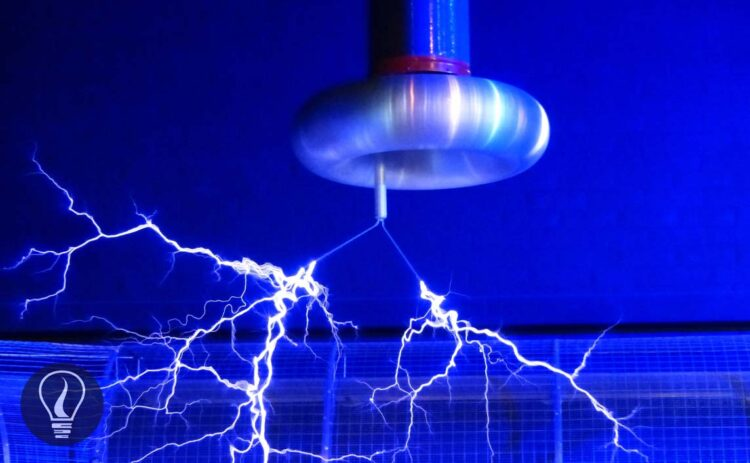More results...

Generic selectors
Exact matches only
Search in title
Search in content
Post Type Selectors

# Electric Charge DensityB.Sc (Hons, USJ) (Polymer Science and Technology, Chemistry, Physics)
Categories
Last Updated On : September 6, 2023
Published Date : September 4, 2023

Electric charge density is measured per unit length, unit area, or unit volume. According to that, the charge density can be a linear charge density, surface charge density, or volume charge density.

## Linear charge density (λ) (m-1C)

Linear charge density is the amount of electric charges per unit length. When we measure the charge density of a wire or any other linear object, we measure the linear charge density. If we assume that there is a Q charge in a linear object with the length of l, the linear charge density can be written as follows.

## Surface charge density (σ) (m-2C)

The surface charge density is the charge per unit area of ​​a plate-like object or a conducting object. If we assume that there is Q charge in an object with the area of A, the surface charge density can be written as follows,

## Volume charge density (ρ) (m-3 C)

Volume charge density is the amount of electric charge per unit volume of a dielectric material. We can only measure the volume charge density for dielectric materials because the conductors remain their charged on their surfaces. If a dielectric object with V volume and contains Q charge, we can find the volume charge density as follows,

## Electric charge density questions and answers

Question 01

The static electric charges contained in a 4 km long high voltage wire are grounded when no current is flowing. In 20 seconds, the static charges in the wire were grounded and the average current flow was 10 A. Find the linear charge density of the wire.

Solution

To find the linear charge density, first, we should find the amount of electric charges in the wire.

Where,

• Q = Amount of electric charges
• I = Current
• t = time

Question 02

What is the surface charge density of a conducting sphere of radius 2 cm when a charge of 4 mC is given?

Solution

Question 03

The radius of a proton is about 1×10-15 m. The charge of a proton is 1.6 ×10-19 C. What is the volume charge density of a proton?

Solution

## Charge density of curved surfaces

Curved surfaces have much higher charge density than flat surfaces. When the radius of the curved surface is decreased, the change density is increased. Tip-like surfaces have the highest charge density. Because a tip is a curved surface with a very small radius. The surface charge density of a curved surface with a radius of r can be written as follows,

The square of the radius of the curved surface is inversely proportional to the surface charge density.## References and Attributes

### Figures:

The cover image was created using an image by Pixabay from Pexels